# Java Program to Check Leap Year

In this tutorial you will learn about the Java Program to Check Leap Year and its application with practical example.

In this tutorial, we will learn to create a Java program that will Check Leap Year using Java programming.

## Prerequisites

Before starting with this tutorial we assume that you are best aware of the following Java programming topics:

• Java Operators.
• Basic Input and Output
• Class and Object.
• Basic Java programming.
• If Else statements
• Loops.

## What is Leap Year?

Logic behind to check whether a year is leap or not is:
Logic of Leap Year:
If the given value is completely divisible by 4, 100 and 400 then it is a leap year.
If the given value is divisible by 4 but not  by 100 then it is a leap year.

Logic for  not a Leap Year:
If the given value is not divisible by 4 then it is not a leap year
If the given value is divisible by 4 and 100 but not divisible by 400 then it is not a leap year.

For example,

• 1221 is not a leap year.
• 2000 is a leap year.
• 1890 is not  a leap year.

## Java Program to Check Leap Year

In this program we will check condition for Leap year or not. We would first declared and initialized the required variables. Next, we would prompt user to input the value for Year. Later we will find entered year is leap year or not.

## Output

Leap Year.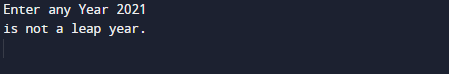Not a Leap Year.In our program, we have first declared and initialized a set variables required in the program.

• year =taking year value from user.
• Flag= for holding true false value.

First of all  we declare variable and take a value from user to check whether a year is leap or not is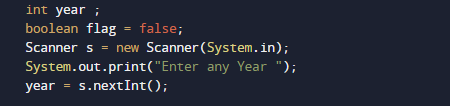then we check if the given value is completely divisible by 4, 100 and 400 then it is a leap year.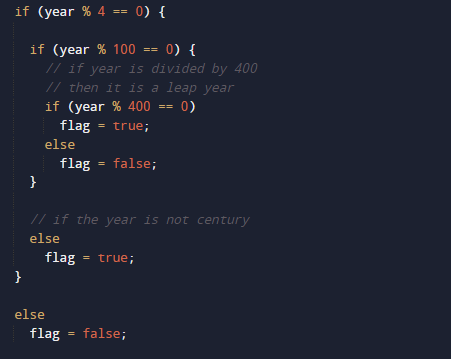If the given value is divisible by 4 but not  by 100 then it is a leap year. As shown in above .So this the logic behind of finding given year is a leap year or not.if value of flag is true then year is leap year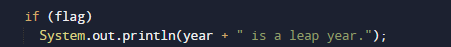and is false than the given year is not.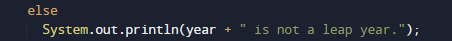In this tutorial we have learn about the Java Program to Check Leap Year and its application with practical example. I hope you will like this tutorial.